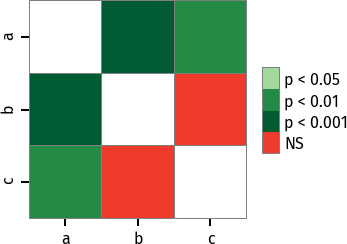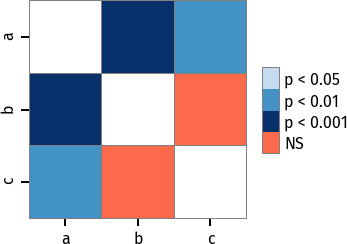# Tutorial¶

## Parametric ANOVA with post hoc tests¶

Here is a simple example of the one-way analysis of variance (ANOVA) with post hoc tests used to compare sepal width means of three groups (three iris species) in iris dataset.

To begin, we will import the dataset using statsmodels `get_rdataset()` method.

```>>> import statsmodels.api as sa
>>> import statsmodels.formula.api as sfa
>>> import scikit_posthocs as sp
>>> df = sa.datasets.get_rdataset('iris').data
Sepal.Length  Sepal.Width  Petal.Length  Petal.Width Species
0           5.1          3.5           1.4          0.2  setosa
1           4.9          3.0           1.4          0.2  setosa
2           4.7          3.2           1.3          0.2  setosa
3           4.6          3.1           1.5          0.2  setosa
4           5.0          3.6           1.4          0.2  setosa
```

Now, we will build a model and run ANOVA using statsmodels `ols()` and `anova_lm()` methods. Columns `Species` and `Sepal.Width` contain independent (predictor) and dependent (response) variable values, correspondingly.

```>>> lm = sfa.ols('Sepal.Width ~ C(Species)', data=df).fit()
>>> anova = sa.stats.anova_lm(lm)
>>> print(anova)
df     sum_sq   mean_sq         F        PR(>F)
C(Species)    2.0  11.344933  5.672467  49.16004  4.492017e-17
Residual    147.0  16.962000  0.115388       NaN           NaN
```

The results tell us that there is a significant difference between groups means (p = 4.49e-17), but does not tell us the exact group pairs which are different in means. To obtain pairwise group differences, we will carry out a posteriori (post hoc) analysis using `scikits-posthocs` package. Student T test applied pairwisely gives us the following p values:

```>>> sp.posthoc_ttest(df, val_col='Sepal.Width', group_col='Species', p_adjust='holm')
setosa    versicolor     virginica
setosa     -1.000000e+00  5.535780e-15  8.492711e-09
versicolor  5.535780e-15 -1.000000e+00  1.819100e-03
virginica   8.492711e-09  1.819100e-03 -1.000000e+00
```

Remember to use a FWER controlling procedure, such as Holm procedure, when making multiple comparisons. As seen from this table, significant differences in group means are obtained for all group pairs.

## Non-parametric ANOVA with post hoc tests¶

If normality and other assumptions are violated, one can use a non-parametric Kruskal-Wallis H test (one-way non-parametric ANOVA) to test if samples came from the same distribution.

Let’s use the same dataset just to demonstrate the procedure. Kruskal-Wallis test is implemented in SciPy package. `scipy.stats.kruskal` method accepts array-like structures, but not DataFrames.

```>>> import scipy.stats as ss
>>> import statsmodels.api as sa
>>> import scikit_posthocs as sp
>>> df = sa.datasets.get_rdataset('iris').data
>>> data = [df.loc[ids, 'Sepal.Width'].values for ids in df.groupby('Species').groups.values()]
```

`data` is a list of 1D arrays containing sepal width values, one array per each species. Now we can run Kruskal-Wallis analysis of variance.

```>>> H, p = ss.kruskal(*data)
>>> p
1.5692820940316782e-14
```

P value tells us we may reject the null hypothesis that the population medians of all of the groups are equal. To learn what groups (species) differ in their medians we need to run post hoc tests. `scikit-posthocs` provides a lot of non-parametric tests mentioned above. Let’s choose Conover’s test.

```>>> sp.posthoc_conover(df, val_col='Sepal.Width', group_col='Species', p_adjust = 'holm')
setosa    versicolor     virginica
setosa     -1.000000e+00  2.278515e-18  1.293888e-10
versicolor  2.278515e-18 -1.000000e+00  1.881294e-03
virginica   1.293888e-10  1.881294e-03 -1.000000e+00
```

Pairwise comparisons show that we may reject the null hypothesis (p < 0.01) for each pair of species and conclude that all groups (species) differ in their sepal widths.

## Block design¶

In block design case, we have a primary factor (e.g. treatment) and a blocking factor (e.g. age or gender). A blocking factor is also called a nuisance factor, and it is usually a source of variability that needs to be accounted for.

An example scenario is testing the effect of four fertilizers on crop yield in four cornfields. We can represent the results with a matrix in which rows correspond to the blocking factor (field) and columns correspond to the primary factor (yield).

The following dataset is artificial and created just for demonstration of the procedure:

```>>> data = np.array([[ 8.82, 11.8 , 10.37, 12.08],
[ 8.92,  9.58, 10.59, 11.89],
[ 8.27, 11.46, 10.24, 11.6 ],
[ 8.83, 13.25,  8.33, 11.51]])
```

First, we need to perform an omnibus test — Friedman rank sum test. It is implemented in `scipy.stats` subpackage:

```>>> import scipy.stats as ss
>>> ss.friedmanchisquare(*data.T)
FriedmanchisquareResult(statistic=8.700000000000003, pvalue=0.03355726870553798)
```

We can reject the null hypothesis that our treatments have the same distribution, because p value is less than 0.05. A number of post hoc tests are available in `scikit-posthocs` package for unreplicated block design data. In the following example, Nemenyi’s test is used:

```>>> import scikit_posthocs as sp
>>> sp.posthoc_nemenyi_friedman(data)
0         1         2         3
0 -1.000000  0.220908  0.823993  0.031375
1  0.220908 -1.000000  0.670273  0.823993
2  0.823993  0.670273 -1.000000  0.220908
3  0.031375  0.823993  0.220908 -1.000000
```

This function returns a DataFrame with p values obtained in pairwise comparisons between all treatments. One can also pass a DataFrame and specify the names of columns containing dependent variable values, blocking and primary factor values. The following code creates a DataFrame with the same data:

```>>> data = pd.DataFrame.from_dict({'blocks': {0: 0, 1: 1, 2: 2, 3: 3, 4: 0, 5: 1, 6:
2, 7: 3, 8: 0, 9: 1, 10: 2, 11: 3, 12: 0, 13: 1, 14: 2, 15: 3}, 'groups': {0:
0, 1: 0, 2: 0, 3: 0, 4: 1, 5: 1, 6: 1, 7: 1, 8: 2, 9: 2, 10: 2, 11: 2, 12: 3,
13: 3, 14: 3, 15: 3}, 'y': {0: 8.82, 1: 8.92, 2: 8.27, 3: 8.83, 4: 11.8, 5:
9.58, 6: 11.46, 7: 13.25, 8: 10.37, 9: 10.59, 10: 10.24, 11: 8.33, 12: 12.08,
13: 11.89, 14: 11.6, 15: 11.51}})
>>> data
blocks  groups      y
0        0       0   8.82
1        1       0   8.92
2        2       0   8.27
3        3       0   8.83
4        0       1  11.80
5        1       1   9.58
6        2       1  11.46
7        3       1  13.25
8        0       2  10.37
9        1       2  10.59
10       2       2  10.24
11       3       2   8.33
12       0       3  12.08
13       1       3  11.89
14       2       3  11.60
15       3       3  11.51
```

This is a melted and ready-to-use DataFrame. Do not forget to pass `melted` argument:

```>>> sp.posthoc_nemenyi_friedman(data, y_col='y', block_col='blocks', group_col='groups', melted=True)
0         1         2         3
0 -1.000000  0.220908  0.823993  0.031375
1  0.220908 -1.000000  0.670273  0.823993
2  0.823993  0.670273 -1.000000  0.220908
3  0.031375  0.823993  0.220908 -1.000000
```

## Data types¶

Internally, `scikit-posthocs` uses NumPy ndarrays and pandas DataFrames to store and process data. Python lists, NumPy ndarrays, and pandas DataFrames are supported as input data types. Below are usage examples of various input data structures.

### Lists and arrays¶

```>>> x = [[1,2,1,3,1,4], [12,3,11,9,3,8,1], [10,22,12,9,8,3]]
>>> # or
>>> x = np.array([[1,2,1,3,1,4], [12,3,11,9,3,8,1], [10,22,12,9,8,3]])
1         2         3
1 -1.000000  0.057606  0.007888
2  0.057606 -1.000000  0.215761
3  0.007888  0.215761 -1.000000
```

You can check how it is processed with a hidden function `__convert_to_df()`:

```>>> sp.__convert_to_df(x)
(    vals  groups
0      1       1
1      2       1
2      1       1
3      3       1
4      1       1
5      4       1
6     12       2
7      3       2
8     11       2
9      9       2
10     3       2
11     8       2
12     1       2
13    10       3
14    22       3
15    12       3
16     9       3
17     8       3
18     3       3, 'vals', 'groups')
```

It returns a tuple of a DataFrame representation and names of the columns containing dependent (`vals`) and independent (`groups`) variable values.

Block design matrix passed as a NumPy ndarray is processed with a hidden `__convert_to_block_df()` function:

```>>> data = np.array([[ 8.82, 11.8 , 10.37, 12.08],
[ 8.92,  9.58, 10.59, 11.89],
[ 8.27, 11.46, 10.24, 11.6 ],
[ 8.83, 13.25,  8.33, 11.51]])
>>> sp.__convert_to_block_df(data)
(    blocks groups      y
0        0      0   8.82
1        1      0   8.92
2        2      0   8.27
3        3      0   8.83
4        0      1  11.80
5        1      1   9.58
6        2      1  11.46
7        3      1  13.25
8        0      2  10.37
9        1      2  10.59
10       2      2  10.24
11       3      2   8.33
12       0      3  12.08
13       1      3  11.89
14       2      3  11.60
15       3      3  11.51, 'y', 'groups', 'blocks')
```

### DataFrames¶

If you are using DataFrames, you need to pass column names containing variable values to a post hoc function:

```>>> import statsmodels.api as sa
>>> import scikit_posthocs as sp
>>> df = sa.datasets.get_rdataset('iris').data
>>> sp.posthoc_conover(df, val_col='Sepal.Width', group_col='Species', p_adjust = 'holm')
```

`val_col` and `group_col` arguments specify the names of the columns containing dependent (response) and independent (grouping) variable values.

## Significance plots¶

P values can be plotted using a heatmap:

```pc = sp.posthoc_conover(x, val_col='values', group_col='groups')
heatmap_args = {'linewidths': 0.25, 'linecolor': '0.5', 'clip_on': False, 'square': True, 'cbar_ax_bbox': [0.80, 0.35, 0.04, 0.3]}
sp.sign_plot(pc, **heatmap_args)
```Custom colormap applied to a plot:

```pc = sp.posthoc_conover(x, val_col='values', group_col='groups')
# Format: diagonal, non-significant, p<0.001, p<0.01, p<0.05
cmap = ['1', '#fb6a4a',  '#08306b',  '#4292c6', '#c6dbef']
heatmap_args = {'cmap': cmap, 'linewidths': 0.25, 'linecolor': '0.5', 'clip_on': False, 'square': True, 'cbar_ax_bbox': [0.80, 0.35, 0.04, 0.3]}
sp.sign_plot(pc, **heatmap_args)
```